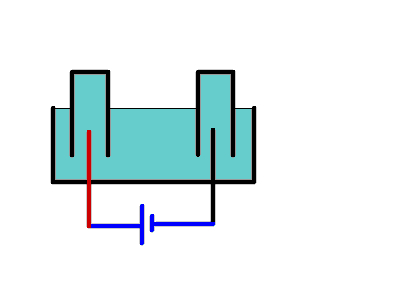## Electrolysis of Water

### Introduction:

Chemical changes (or chemical reactions) can be of two general types:

1. Analysis
and
2. Synthesis.

Analysis are chemical changes used to anayze or to break down a substance.

For example, breaking down compounds into elements.

Synthesis are chemical reactions used to put  simple substances together into more complex ones.

For example, bonding elements into compounds.

If we wanted to analyze water, and take it apart to find what elements it is made up of, we use a special type of analysis that utilizes electricity.

This type of analysis is called Electrolysis

The set up is simple: (see diagram )WARNING: Please don't try this demonstration at home or on your own without the direct supervision of an adult.

A bit a of acid was added to the water.  The reason for adding acid to the water is to speed up the reaction.

A chemical used in chemical reactions to speed up the process (without affecting the final product) is called a catalyst.

There are two inverted jars in the water tank.

Each jar has a piece of wire in it connected to a battery (or a direct current power source).

One jar has a positive electrode in it, the other jar has a negative electrode placed in it.

### Observations:

1. When the power is turned on, bubbles of gas start forming at each electrode.

2. We note that about twice more gas is being formed at the negative electrode than it is formed at the positive electrode.

3. When a good volume of gas was collected in the jars,  the power is turned off and each gas is tested using the flame tests.

4. Using a burning splint the gas at the negative electrode is tested.   This produces a "pop" sound, and the gas burns with a blue flame.

5. Using a glowing splint the gas at the positive electrode is tested.  The glowing splint bursts into flames.

### Notes:

1.  The gas collected at the negative electrode is HYDROGEN.

2. The gas collected at the positive electrodes is OXYGEN.

3. The proportion of hydrogen to volume in water (by volume) is two parts of hydrogen are present for every part of oxygen.

### Inferences:

1. We can say that regardless of the amount of water taken into consideration, (ONE DROP, ONE GLASS, ONE LAKE...) the relative proportions of the elements that make up water (hydrogen and Oxygen) are  Hydrogen : Oxygen = 2 : 1

2. This means that the chemical formula for water is always H2O.

### Conclusions:

The fact that elements combine in definite proportions was first proposed by Dalton.

In his Law of Definitive Proportions he states that when elements combine to form compounds, they do so in definite, small number ratios.